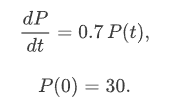# Calculation

Course subject(s) 1. Introducing Mathematical Modelling

In the previous section you have derived the differential equation and the intitial condition:Is there a function P(t) that satisfies this initial value problem?